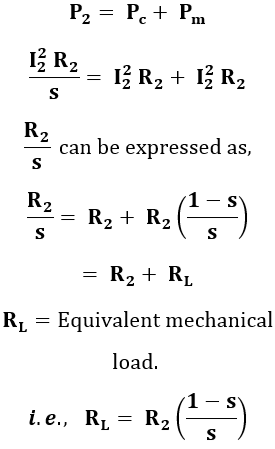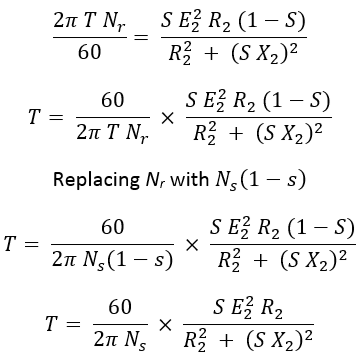# Equivalent circuit of an Induction Motor

An induction motor is an asynchronous motor i.e., its speed change with a change in load. It always runs on a lagging power factor. The principle of working of an induction motor to similar to the transformer i.e., electromagnetic induction.

The equivalent circuit of an induction motor is similar to a transformer equivalent circuit because the energy is transferred from stator to rotor is essential as a transformer operation from primary to the secondary winding.

An equivalent circuit enables the performance characteristics of the induction motor. The data obtained from the equivalent circuit can be used to calculate efficiency, torque, losses, rotor output, etc. All per phase quantities are used in representing the equivalent circuit.

## Equivalent Circuit of induction Motor :

#### From the above diagram, the rotor current I2 is given by,Here we know that the rotor input, P2 is the sum of rotor copper losses Pc and mechanical power developed Pm. Thus it is possible to represent the electrical equivalent of mechanical power developed as follows,To show the equivalent mechanical load (mechanical power conversion) in the rotor circuit. The motor equivalent circuit can be modified as,

Now transfer the rotor side parameters to the stator side. While shifting the rotor side parameters towards the stator side we have to divide it by the value "K" (Where K = Ratio of the effective rotor to stator turns per phase) except the rotor current where it is multiplied by "K". When the rotor parameters are shifted they can be represented as,

## Calculation of Rotor Output and Torque Using the Equivalent Circuit :

#### By substituting the value of I2 in equation (1) we get,We know that the rotating magnetic field of the induction motor produced by the stator winding rotates at synchronous speed Ns. Then the torque applied on the rotor due to the stator rotating magnetic field will be T. Now, the power transferred from stator to rotor is given by,Equating the equations 2 & 3 we get,Therefore, the torque equation is derived from the equivalent circuit and various performance calculations can be performed from the above equations.

Do not enter any spam links and messages